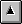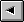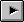## HelpUntil includes a built-in help facility. Simply type:

```      help any_word
```
to display the help entry for any_word. The help text is contained in the file HELP.APP. This file is a simple Ascii file. Each entry begins in column 1. Lines with leading spaces (tab characters too) are considered part of the entry. The first blank line is considered end of file. Sample help entries are shown below:
```@  ( addr --- value)
Fetch the contents of the integer at addr.
!  ( n addr --- )
```
These help entries set up entries for @ and !.

An alternative approach is compiling the help information into the executable image. This approach was not taken with Until for the following reasons:

• The executable file size would be significantly larger.
• The messages are fixed at compile time.
• The help file approach allows the Until help facility to be modified for each application.

The help system is designed to be easy to maintain entries. The data is contained in an Ascii file. It can be edited to match any application that contains Until. See Write Your Own Programming Language Using C++; it includes an entire chapter on HELP.

## Vectored WordsSeveral I/O words are vectored in Until by default, the C primitives READ_WA() and FREAD_WA(). The vectoring handles the switch from reading the keyboard to reading a file and back.

The following words may be vectored in Until:

• .
• ."
• ;
• cr
• emit
• key
• number
• space
• spaces
• ?terminal
• type

The default Until configuration does not vector any of these words. Define the symbol VECTORED_IO and recompile Until to enable the vectored I/O words.

There is a slight performance penalty for using vectored I/O. The extra level of call indirection causes an extra C call each time any vectored word is called. This is the reason for defaulting to non-vectored I/O.

The I/O vectoring, as currently implemented, is at the C level. You cannot vector high level Forth words. The present scheme provides the hooks. You provide the C functions via primitive words for changing the contents of the vectors and the C functions to call via the vectors. The following steps describe the steps to add your own vector for spaces:

• Write the new C function to replace the default spaces(). We'll call it new_spaces() for this example.
• Write a primitive, set_new_spaces() to change insert the C function address of new_spaces into the vector for spaces.
• Bind the address of set_new_spaces() to the Forth word NEW-SPACES by adding an entry in USER.C.
• Write a primitive, restore_spaces() to restore the original vector contents.
• Bind the address of restore_spaces() to the Forth word RESTORE-SPACES by adding an entry in USER.C.
• Recompile Until with VECTORED_IO defined in COMPILER.H.

This scheme provides the user with a way to both set and restore the vector for spaces. Adding new vectors for any of the words identified in the word list above can be done using the same steps listed here for spaces().

Vectoring via high level words is not supported in Until in this release. The hooks are there now. Expect vectored execution capabilities to be increased in future releases.

Add discussion of low level C I/O vectoring.

## Floating Point WordsThe present floating point package is a minimal implementation. It provides a way to input floating point numbers, manipulate the floating point stack, and output floating point numbers. Any of the C floating point library functions can be easily built upon with the basic capabilities included.

The floating point package uses a separate floating point stack. Floating point numbers all reside on the floating point stack. Addresses and true/false flags all reside on the parameter stack.

f!
"f-store". Store the floating point value, fn, at address specified by addr.
```      f# 3.1417 pi f!
```
moves 3.1417 into the floating point variable pi.
f#
( --- f# )
"f-number". Interpret the next number in the input in the input stream as a floating point number.
```     f# 123.45  float-foo f!
```

f*
( f1 f2 --- f-product )
"f-star". Multiply two floating point numbers leaving the result on the floating point stack.
```     f# 2.0  f# 2.0 f* f.
4.00
```
multiplies 2.0 by 2.0 and prints the result on the screen.
f+
( f1 f2 --- f-sum )
"f-plus". Add f1 to f2. The sum is placed on the top of the floating point stack.
```      f# 1.0 f# 2.0 +
```
The result, 3.0, is left on the top of the floating point stack.
f-
( f1 f2 --- f3 )
"f-minus". Subtract f2 from f1 and place the result on the top of the floating point stack. (f1-f2=f3).
```      f# 2 f# 1 f-
```
The result, 1.0, is left on the stack.
f.
( fn --- )
"f-dot". Display the number at the top of the floating point stack to the screen. This is a stack destructive operation.
f.s
( ? --- ? )
"f-dot-s". Non-destructive floating point stack display to the screen.
f/
( f1 f2 --- fquotent )
"f-divide". Divide f1 by f2. (f1/f2).
```      4 2 /
```
Leaves 2.0 on the top of the floating point stack. There is no remainder.
```      f# 9 f# 2 f/
```
computes to 4.50.
f<
( f1 f2 --- tf )
"f-less-than". Compare the two numbers on the top of the stack and return TRUE or FALSE.
```      f# 5 f# 6 f<          ( RETURNS true  )
f# 5 f# 5 f<          ( RETURNS false )
f# 6 f# 5 f<          ( RETURNS false )
```

f=
( f1 f2 --- tf )
"f-equal". Compare the top two values on the stack for equality.
```      f# 5 f# 6 f=         ( returns false )
f# 5 f# 5 f=         ( returns true  )
f# 6 f# 5 f=         ( returns false )
```

f>
( f1 f2 --- tf )
"f-greater-than". Compare the top two floating point stack entries. (f1>f2).
```      f# 5 f# 6 f>          ( returns false )
f# 5 f# 5 f>          ( returns false )
f# 6 f# 5 f>          ( returns true  )
```

>float
( n --- f1 )
"to-float". Convert an integer value on the parameter stack to a floating point number on the floating point stack.
```     1 >float
```
leaves 1.0 on the floating point stack.
f@
"f-fetch". Fetch the floating point value from address and place on the top of the floating point stack.
```      ftrash f@
```
retrieves the value stored at ftrash and places it on the top of the floating point stack.
fconstant
( --- fn )
"f-constant". Create a floating point constant. The compile time stack comment is ( fn --- ). Typical usage is:
```      f# 3.1416 fconstant pi
```
When pi is referenced, the floating point value 3.1416 is pushed onto the floating point stack:
```      pi    ( --- 3.1416 )
```

fdrop
( f1 --- )
"f-drop". Remove the top number from the floating point stack.
fdup
( f1 --- f1 f1 )
"f-dup". Duplicate the number on the top of the floating point stack.
```      f# 1.0 f# 1.0 fdup
```
Results in two 1.0s on the floating point stack.
float>
( f1 --- n )
"from-float". Convert a floating point number to integer.
```     f# 123.456 float>
```
leaves 123 on the parameter stack.
fover
( f1 f2 --- f1 f2 f1 )
"f-over". Copy f1 to the top of the floating point stack.
```      f# 1 f# 2 fover
```
results in ( 1.0 2.0 1.0 ).
frot
( f1 f2 f3 --- f2 f3 f1 )
"f-r-o-t". Rotate the third value on the floating point stack to the top. The other values are pushed down one cell.
```      f# 1 f# 2.0 f# 3.0 frot
```
results in (2.0 3.0 1.0).
fswap
( f1 f2 --- f2 f1 )
"f-swap". Swap the top two entries on the top of the floating point stack.
```      f# 1.0 f# 2.0 fswap
```
results in the top stack entry being 2.0 and the second entry being 1.0.
fvariable
( --- 'fvariable )
"f-variable". At compile time, creates a floating point variable:
```      fvariable ftrash
```
The compile time stack comment for variable is ( --- ). At run time, push the address of the floating point variable onto the stack:
```      ftrash    ( --- addr.of.trash )
```

## Floating Point ExamplesTBSTable of ContentsNext Section